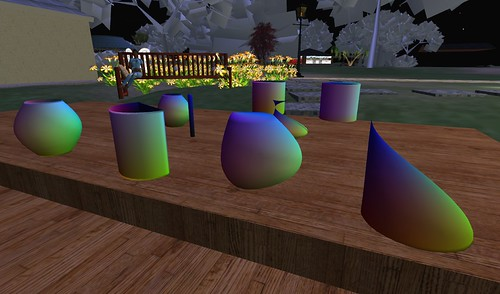## Making sculpties with code

I finally got around to doing this!Here I am sitting in my place in Inworldz (where the texture uploads are free, heh heh), trying out the sculpt-maps made by this Python program that I wrote that makes sculpt maps.

My favorite so far is the pointy one on the right there, which resulted from a couple of bugs in the program. :)

(And yeah, the Linden trees are being mysteriously grey again.)

Here are a couple of the sculpt textures themselves:

The fun pointy one:Another odd bug-generated one:And the very symmetrical one on the left, once all the bugs were out:And finally (Secrets of the Ages Revealed!) the Python code itself:

```import sys
import math
from PIL import Image

X = 64
Y = X

fname = sys.argv if len(sys.argv)>1 else "sculpt.png"

def getR(x,y):
return(      math.sin( y * 2 * math.pi )  *
(( math.sin( x * math.pi ) + 1 ) / 2)         )

def getG(x,y):
return(      math.cos( y * 2 * math.pi )  *
(( math.sin( x * math.pi ) + 1 ) / 2)        )

def getB(x,y):
return( x*2 - 1  )

def gogogo():
ary = []
for x in range(X):
for y in range(Y):
ary.append( (
127+int(127.0* getR(float(x)/X,float(y)/Y)),
127+int(127.0* getG(float(x)/X,float(y)/Y)),
127+int(127.0* getB(float(x)/X,float(y)/Y))  ) )
mode = "RGB"
size = (X,Y)
im = Image.new(mode,size)
im.putdata(ary)
im.save(fname,"PNG")

gogogo()```

I trust it’s obvious how to use it.

Hahaha, sorry! Couldn’t resist. :)

To use it, well, first install Python and also the Python Imaging Library, then create a “mksc.py” or whatever you want to call it from the code above. Do “python mksc.py vase.png”, and it will create a file “vase.png” which you can upload and use as a sculpt texture to make the symmetrical thing on the left.

Then edit your mksc.py to change the getR, getG, and getB functions to something else, and do “python mksc.py somethingelse.png”, and upload somethingelse.png and use it as a sculpt texture, and see what happens!

For best results, getR, getG, and getB should all expect to get two floating point numbers between zero and one as input, and should return a single floating point number between negative one and one as output.

And there ya go! :)

Oh, and: you may find that the textures work best if uploaded with lossless compression (probably a checkbox on your image-upload panel), and that it’s more obvious what’s going on it you use the “torus” stitching type (in the edit panel right under where you put the sculpt texture) rather than the default “sphere” type. P’haps…

### 8 Responses

1. Way cool! I know next to nothing about Python, but I really like the mathematical approach and hope to give it a try sometime…

• Well, ty! The program is simple and should translate to any other language without much trouble.

As with the Thingmaker, I’m much better at making programs that make things than I am at making things directly myself. Math makes things easy. :)